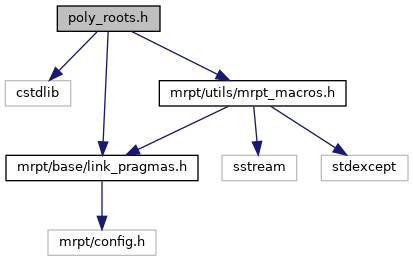Main MRPT website > C++ reference for MRPT 1.5.7
poly_roots.h File Reference
`#include <cstdlib>`
`#include <mrpt/utils/mrpt_macros.h>`
`#include <mrpt/base/link_pragmas.h>`
Include dependency graph for poly_roots.h:This graph shows which files directly or indirectly include this file:Go to the source code of this file.

## Namespaces

mrpt
This is the global namespace for all Mobile Robot Programming Toolkit (MRPT) libraries.

mrpt::math
This base provides a set of functions for maths stuff.

## Functions

int BASE_IMPEXP mrpt::math::solve_poly3 (double *x, double a, double b, double c) MRPT_NO_THROWS
Solves cubic equation `x^3 + a*x^2 + b*x + c = 0`. More...

int BASE_IMPEXP mrpt::math::solve_poly4 (double *x, double a, double b, double c, double d) MRPT_NO_THROWS
Solves quartic equation `x^4 + a*x^3 + b*x^2 + c*x + d = 0` by Dekart-Euler method. More...

int BASE_IMPEXP mrpt::math::solve_poly5 (double *x, double a, double b, double c, double d, double e) MRPT_NO_THROWS
Solves equation `x^5 + a*x^4 + b*x^3 + c*x^2 + d*x + e = 0`. More...

int BASE_IMPEXP mrpt::math::solve_poly4Bi (double *x, double b, double d) MRPT_NO_THROWS
Solve equation x^4 + b*x^2 + d = 0. More...

int BASE_IMPEXP mrpt::math::solve_poly4De (double *x, double b, double c, double d) MRPT_NO_THROWS
Solve equation x^4 + b*x^2 + c*x + d = 0. More...

int BASE_IMPEXP mrpt::math::solve_poly2 (double a, double b, double c, double &r1, double &r2) MRPT_NO_THROWS
Solves equation `a*x^2 + b*x + c = 0`. More...

 Page generated by Doxygen 1.8.14 for MRPT 1.5.7 Git: 8277875f6 Mon Jun 11 02:47:32 2018 +0200 at lun oct 28 01:50:49 CET 2019